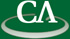## Faster Grobner Basis Computation

### Jennifer de Kleine, SFU

```We have done a modular design and implementation of Buchberger's
algorithm in Maple V Release 5.  In our method we compute Grobner
bases modulo batches of primes and then reconstruct the rational
answer using Chinese remaindering and rational reconstruction.

The bottleneck of this method is the division algorithm, in particular
the monomial arithmetic and vector operations in Zp_1 x ... x Zp_b.
We have now coded these critical operations in C and have linked the
external C subroutines to Maple.  In our polynomial representation we
store the coefficients in a 2-dimensional array of hardware floats and
the monomial exponent vectors in a 2-dimensional array of integers.
We are using 8 decimal digit primes and the modular arithmetic is done
using floating point arithmetic.  We have achieved significant
run-time improvements.
```